# Frank solutions for Class 9 Maths ICSE chapter 25 - Surface Areas and Volume of Solids [Latest edition]

#### Chapters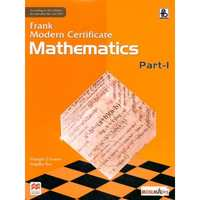## Chapter 25: Surface Areas and Volume of Solids

Exercise 25.1Exercise 25.2Exercise 25.3
Exercise 25.1

### Frank solutions for Class 9 Maths ICSE Chapter 25 Surface Areas and Volume of Solids Exercise 25.1

Exercise 25.1 | Q 1

The volume of a cube is 1331 cm3. Find its total surface area.

Exercise 25.1 | Q 2

The total surface area of a cube is 864 cm2. Find its volume.

Exercise 25.1 | Q 3

The length, breadth, and height of a rectangular solid are in the ratio 6 : 4 :3. If the total surface area is 1728 cm2. Find its dimensions.

Exercise 25.1 | Q 4

Find the volume of a cube whose diagonals is sqrt(48)"cm".

Exercise 25.1 | Q 5

The length and breadth of a cuboid are 20 cm and 15 cm respectively. If its volume is 2400 cm3, find its height.

Exercise 25.1 | Q 6

Find the volume of a cuboid whose diagonal is 3sqrt(29)"cm" when its length, breadth and height are in the ratio 2 : 3 : 4.

Exercise 25.1 | Q 7

The total surface area of a cube is 294cm2. Find its volume.

Exercise 25.1 | Q 8

The total surface area of a cuboid is 46m2. If its height is 1m and breadth 3m, find its length and volume.

Exercise 25.1 | Q 10

The length breadth and height of a cuboid are in the ratio of 3 : 3 : 4. Find its volume in m3 if its diagonal is 5sqrt(34)"cm".

Exercise 25.1 | Q 11

A square plate of side 'x' cm is 4 mm thick. If its volume is 1440 cm3; find the value of 'x'.

Exercise 25.1 | Q 12

A room is 22m long, 15m broad and 6m high. Find the area of its four walls and the cost of painting including doors and windows at the rate of Rs.12per m2.

Exercise 25.1 | Q 13

A cuboid is 25cm long, 15cm board and 9cm high. Find the whole surface of a cube having its volume equal to that of the cuboid.

Exercise 25.1 | Q 14

The square on the diagonal of a cube has an area of 441 cm2. Find the length of the side and total surface area of the cube.

Exercise 25.1 | Q 15

Three metal cubes with edges 6cm, 8cm and 10cm respectively are melted together and formed into a single cube. Find the diagonal of this cube.

Exercise 25.1 | Q 16

Three cubes of sides x cm, 8cm and 10cm respectively are melted and formed into a single cube of edge 12cm, Find 'x'.

Exercise 25.1 | Q 17

Three equal cubes of sides 5cm each are placed to form a cuboid. Find the volume and the total surface area of the cuboid.

Exercise 25.1 | Q 21

Three equal cubes are placed adjacently in a row. Find the ratio of the total surface area of the resulting cuboid to that of the sum of the total surface areas of the three cubes.

Exercise 25.1 | Q 22

A metal cube of side 4 cm is immersed in a water tank. The length and breadth of the tank are 8 cm and 4 cm respectively. Find the rise in level of the water.

Exercise 25.1 | Q 23

A metal piece 6 cm long, 5 cm broad and x cm , high is dropped in a glass box containing water. The dimensions of the base of the glass box are 18 cm by 8 cm and the rise in water level is 0.5 cm. Find x.

Exercise 25.1 | Q 24

A closed box is made of wood 5 mm thick. The external length, breadth and height of the box are 21 cm, 13 cm and 11 cm respectively. Find the volume of the wood used in making the box.

Exercise 25.1 | Q 26

A room is 5m long, 2m broad and 4m high. Calculate the number of persons it can accommodate if each person needs 0.16m3 of air.

Exercise 25.1 | Q 27

375 persons can be accommodated in a room whose dimensions are in the ratio of  6 : 4 : 1. Calculate the area of the four walls of the room if the each person consumes 64m3 of air.

Exercise 25.1 | Q 28

A class room is 7m long, 6m wide and 4m high. It has two doors each of 3m x 1.4m and six windows each of 2m x 1m. The interior walls are to be coloured at the rate of Rs.15per m2. Find the cost of colouring the walls.

Exercise 25.1 | Q 30

The cost of papering the four walls of a room at Rs 1 per m2 is Rs. 210. The height of the room is 5 m. Find the length and the breadth of the room if they are in the ratio 5:2.

Exercise 25.1 | Q 32

Find the volume of wood used in making a closed box 22 cm by 18 cm by 14 cm, using a 1 cm thick wood. Also, find the cost of wood required to make the box at the rate of Rs. 5 per cm³ How many cubes of side 2 cm can be placed in the box?

Exercise 25.1 | Q 33

The length of a cold storage is double its breadth. Its height is 3m. The area of its four walls including doors is 108m2. Find its volume.

Exercise 25.1 | Q 34

A metallic sheet is of the rectangular shape with dimensions 48cm x 36cm. From each one of its corners, a square of 8cm is cutoff. An open box is made of the remaining sheet. Find the volume of the box.

Exercise 25.1 | Q 35

The area of a playground is 4800m2. Find the cost of covering it with gravel 2.5cm deep, if the gravel costs Rs.7.25 per m3.

Exercise 25.1 | Q 36

A rectangular container has base with dimensions 6 cm x 9 cm. A cube of edge 3 cm is placed in the container and then sufficient water is filled into it so that the cube is just submerged. Find the fall in the level of the water in the container, when the cube is removed.

Exercise 25.1 | Q 39

The base of a rectangular container is a square of side 12 cm. This container holds water up to 2 cm from the top. When a cube is placed in the water and is completely submerged, the water rises to the top and 224 cm3 of water overflows. Find the volume and surface area of the cube.

Exercise 25.2

### Frank solutions for Class 9 Maths ICSE Chapter 25 Surface Areas and Volume of Solids Exercise 25.2

Exercise 25.2 | Q 1.1

Find the lateral surface area, total surface area and the volume of the following cylinders: Radius = 4.2cm, Height = 12cm

Exercise 25.2 | Q 1.2

Find the lateral surface area, total surface area and the volume of the following cylinders: Diameter = 10m, High = 7m

Exercise 25.2 | Q 2

A cylinder has a diameter 20cm. The area of the curved surface is 1100cm2. Find the height and volume of the cylinder.

Exercise 25.2 | Q 3

Find the volume of a cylinder which has a base diameter cm and height 24cm.

Exercise 25.2 | Q 4

The total surface area of a cylinder is 264m2. Find its volume if its height is 5 more than its radius.

Exercise 25.2 | Q 5

The curved surface area of a cylinder is 198cm2 and its base has diameter 21cm. Find the height and the volume of the cylinder.

Exercise 25.2 | Q 6

The volume of a solid cylinder is 7700cm3. Find its height and total surface area if the diameter of its base is 35cm.

Exercise 25.2 | Q 7

The total surface area of a cylinder is 3872cm2. Find its height and volume if the circumference of the base is 88cm.

Exercise 25.2 | Q 9

The radius of the base of a right circular cylinder is doubled and the height is halved. What is the ratio of volume of the new cylinder to that of the original cylinder?

Exercise 25.2 | Q 10

The radius of the base of a right circular cylinder is tripled and the height is doubled. What is the ratio of volume of the new cylinder to that of the original cylinder?

Exercise 25.2 | Q 11

The volume of a cylinder of height 8cm is 392π cm3. Find its lateral surface area and its total surface area.

Exercise 25.2 | Q 12

Find the length of 22 kg copper wire of diameter 0.8 cm, if the weight of 1 cm3 copper is 4.2 g.

Exercise 25.2 | Q 13

Find the length of a solid cylinder of diameter 4 cm when recast into a hollow cylinder of outer diameter 10 cm, thickness 0.25 cm and length 21 cm? Give your answer correct to two decimal places.

Exercise 25.2 | Q 15

A hollow garden roller, 1 m wide with outside diameter of 30 cm, is made of 2 cm thick iron. Find the volume of the iron. If the roller rolls without sliding for 6 seconds at the rate of 8 complete rounds per second, find the distance travelled and the area covered by the roller in 6 seconds.

Exercise 25.2 | Q 16

A rectangular metal sheet 36cm x 20cm can be formed into a right circular cylinder, either by rolling its length or by rolling along its breadth. Find the ratio of the volumes of the two cylinders thus formed.

Exercise 25.2 | Q 17

Find the ratio of the volumes of the two cylinders formed by rolling an iron sheet 2.2m x 1.m ether along its length or by rolling along its breadth.

Exercise 25.2 | Q 18

A rectangular strip 36cm x 22cm is rotated about the longer side. Find the volume and the total surface area of the cylinder formed.

Exercise 25.2 | Q 19

The height of a circular cylinder is 4.2cm. There times the sum of the areas of its two circular faces is twice the area of the curved surface. Find the volume of the cylinder correct to 1 decimal place.

Exercise 25.2 | Q 20

How many cubic meters of earth must be dug out to sink a well 42m deep and 14m in diameter? Find the cost of plastering the inside walls of the well at Rs.15per m2.

Exercise 25.2 | Q 21

A well with 14m diameter is dug 21m deep. The earth taken out of it has been evenly spread all around it to a width of 14m to form an embankment. Find the height of the embankment.

Exercise 25.2 | Q 23

A well with 6 m diameter is dug. The earth taken out of it is spread uniformly all around it to a width of 2 m to form an embankment of height 2.25 m. Find the depth of the well.

Exercise 25.2 | Q 25

A cylindrical container with internal diameter of its base 20 cm, contains water upto a height of 14 cm. Find the area of the wet surface of the cylinder.

Exercise 25.2 | Q 26

The radius of a solid cylinder decreases by 10% and its height increases by 20%. Find the change in percentage of its volume and curved surface area

Exercise 25.2 | Q 27

From a tap of inner radius 0.80 cm, water flows at the rate of 7 m/s. Find the volume in litres of water delivered by the pipe in 75 minutes.

Exercise 25.2 | Q 28

A cylindrical water tank has a diameter 4 m and is 6 m high. Water is flowing into it from a cylindrical pipe of diameter 4 cm at the rate of 10 m/s. In how much time the tank will be filled?

Exercise 25.2 | Q 29

The difference between the outer and inner curved surface area of a hollow cylinder is 264 cm2. If its height is 14 cm and the volume of the material in it is 1980 cm3, find its total surface area.

Exercise 25.2 | Q 30

The sum of the height and the radius of a cylinder is 28 cm and its total surface area is 616 cm2, find the volume of the cylinder.

Exercise 25.2 | Q 31

A cylindrical tube, open at both ends, is made of metal. The bore (internal diameter) of the tube is 10.4 cm and its length is 25 cm. The thickness of the metal is 8 mm everywhere. Calculate the volume of the metal. Also, find the weight of the tube if 1 cm3 of the metal weighs 1.42 g.

Exercise 25.3

### Frank solutions for Class 9 Maths ICSE Chapter 25 Surface Areas and Volume of Solids Exercise 25.3

Exercise 25.3 | Q 1.1

The cross section of a piece of metal 2 m in length is shown. Calculate the area of cross section.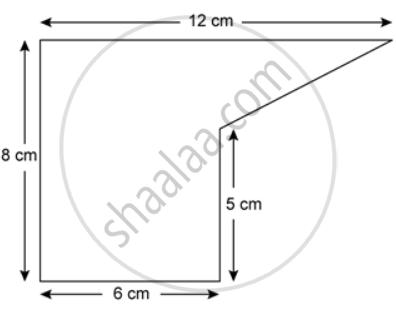Exercise 25.3 | Q 1.2

The cross section of a piece of metal 2 m in length
is shown. Calculate the volume of the piece of metal.Exercise 25.3 | Q 2

The figure represents the cross section of a swimming pool 10 m broad, 2 m deep at one end, 3 m deep at the other end. Calculate the volume of water it will hold when full, given that its length is 40 m.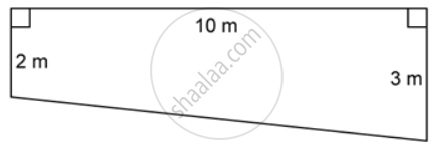Exercise 25.3 | Q 3.1

The given figure is a cross -section of a victory stand used in sports. All measurements are in centimetres. Assume all angles in the figure are right angles. If the width of the stand is 60 cm, find The space it occupies in cm3.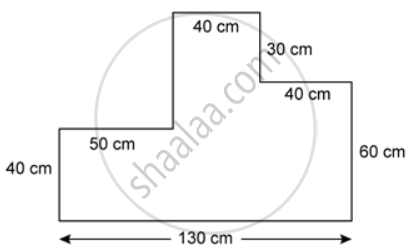Exercise 25.3 | Q 3.2

The given figure is a cross -section of a victory stand used in sports. All measurements are in centimetres. Assume all angles in the figure are right angles. If the width of the stand is 60 cm, find The total surface area in m2.

Exercise 25.3 | Q 4

Water flows at the rate of 1.5 meters per second through a pipe with area of cross section 2.5 cm2 into a rectangular water tank of length 90 cm and breadth 50 cm. Find the rise in water level in the tank after 4 minutes.

Exercise 25.3 | Q 5

A swimming pool is 50 m long and 15 m wide. Its shallow and deep ends are 1.5 m and 4.5 m respectively. If the bottom of the pool slopes uniformly, find the amount of water in kilolitres required to fill the pool (1 m3 = 1000 liters).

Exercise 25.3 | Q 6

Rain falls on a rectangular roof 28 m by 9 m and the water flows into a tank of dimensions 90 m by 70 cm by 84 cm. How much rainfall will fill the tank completely?

Exercise 25.3 | Q 7

The area of cross section of a pipe is 5.4 square cm and water is pumped out of it at the rate of 27 km per hour. Find, in litres, the volume of water which flows out of the pipe in 2 minutes.

Exercise 25.3 | Q 8.1

The figure shows the cross section of 0.2 m a concrete wall to be constructed. It is 0.2 m wide at the top, 2.0 m wide at the bottom and its height is 4.0 m, and its length is 40 m. Calculate the cross sectional area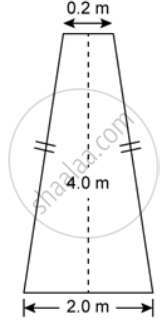Exercise 25.3 | Q 8.2

The figure shows the cross section of 0.2 m a concrete wall to be constructed. It is 0.2 m wide at the top, 2.0 m wide at the bottom and its height is 4.0 m, and its length is 40 m. Calculate the volume of the concrete in the wallExercise 25.3 | Q 8.3

The figure shows the cross section of 0.2 m a concrete wall to be constructed. It is 0.2 m wide at the top, 2.0 m wide at the bottom and its height is 4.0 m, and its length is 40 m. If the whole wall is to be painted, find the cost of painting it at 2.50 per sq m.Exercise 25.3 | Q 9.1

The cross section of a tunnel perpendicular to its length is a trapezium ABCD as shown in the figure. AM = BN; AB = 4.4 m, CD = 3 m The height of a tunnel is 2.4 m. The tunnel is 5.4 m long. Calculate the cost of painting the internal surface of the tunnel (excluding the floor) at the rate of Rs. 5 per m2.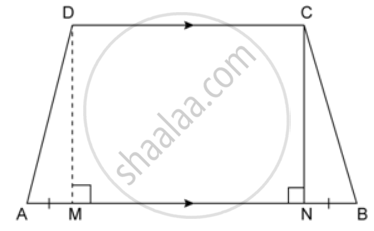Exercise 25.3 | Q 9.2

The cross section of a tunnel perpendicular to its length is a trapezium ABCD as shown in the figure. AM = BN; AB = 4.4 m, CD = 3 m The height of a tunnel is 2.4 m. The tunnel is 5.4 m long. Calculate the cost of flooring at the rate of Rs.2. 5 per m2.Exercise 25.3 | Q 10.1

ABCDE is the end view of a factory shed which is 50 m long. The roofing of the shed consists of asbestos sheets as shown in the figure. The two ends of the shed are completely closed by brick walls.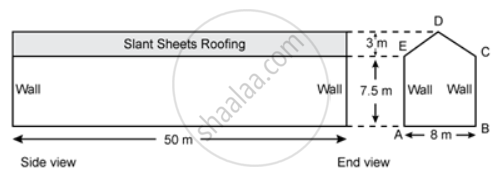Calculate the total volume content of the shed.

Exercise 25.3 | Q 10.2

ABCDE is the end view of a factory shed which is 50 m long. The roofing of the shed consists of asbestos sheets as shown in the figure. The two ends of the shed are completely closed by brick walls.If the cost of asbestos sheet roofing is Rs. 20 per m2, find the cost of roofing.

Exercise 25.3 | Q 10.3

ABCDE is the end view of a factory shed which is 50 m long. The roofing of the shed consists of asbestos sheets as shown in the figure. The two ends of the shed are completely closed by brick walls.Find the total surface area (including roofing) of the shed.

Exercise 25.3 | Q 11

The cross section of a swimming pool is a trapezium whose shallow and deep ends are 1 m and 3 m respectively. If the length of the pool is 50 m and its width is 1.5 m, calculate the volume of water it holds.

Exercise 25.3 | Q 12

A hose-pipe of cross section area 3 cm2 delivers 1800 liters of water in 10 minutes. Find the speed of water in km/h through the pipe.

Exercise 25.3 | Q 13

The cross section of a canal is a trapezium with the base length of 3 m and the top length of 5 m. It is 2 m deep and 400 m long. Calculate the volume of water it holds.

Exercise 25.3 | Q 14

A rectangular water tank measuring 8mx6mx 4 cm is filled from a pipe of cross sectional area 1.5 cm2, the water emerging at 10 m/s. How long does it take to fill the tank?

Exercise 25.3 | Q 15

How many liters of water will flow out of a pipe having a cross sectional area 6 cm2 in one hour, if the speed of water in the pipe is 30 cm/sec?

## Chapter 25: Surface Areas and Volume of Solids

Exercise 25.1Exercise 25.2Exercise 25.3## Frank solutions for Class 9 Maths ICSE chapter 25 - Surface Areas and Volume of Solids

Frank solutions for Class 9 Maths ICSE chapter 25 (Surface Areas and Volume of Solids) include all questions with solution and detail explanation. This will clear students doubts about any question and improve application skills while preparing for board exams. The detailed, step-by-step solutions will help you understand the concepts better and clear your confusions, if any. Shaalaa.com has the CISCE Class 9 Maths ICSE solutions in a manner that help students grasp basic concepts better and faster.

Further, we at Shaalaa.com provide such solutions so that students can prepare for written exams. Frank textbook solutions can be a core help for self-study and acts as a perfect self-help guidance for students.

Concepts covered in Class 9 Maths ICSE chapter 25 Surface Areas and Volume of Solids are Introduction of Solids, Surface Area of a Cube, Cost of an Article, Cross Section of Solid Shapes, Flow of Water ( or any other liquid ), Surface Area of Cylinder, Surface Area of a Cuboid.

Using Frank Class 9 solutions Surface Areas and Volume of Solids exercise by students are an easy way to prepare for the exams, as they involve solutions arranged chapter-wise also page wise. The questions involved in Frank Solutions are important questions that can be asked in the final exam. Maximum students of CISCE Class 9 prefer Frank Textbook Solutions to score more in exam.

Get the free view of chapter 25 Surface Areas and Volume of Solids Class 9 extra questions for Class 9 Maths ICSE and can use Shaalaa.com to keep it handy for your exam preparation